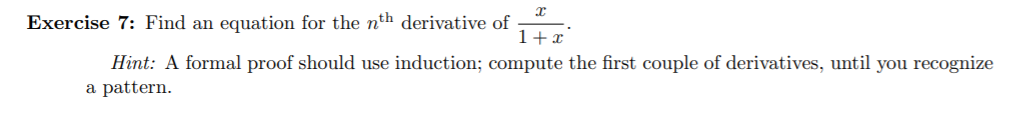# Exercise 7: Find an equation for the nth derivative of 1+r Hint: A formal proof should use induction; compute the first couple of derivatives, until you recognize a pattern

Questionhelp_outlineImage TranscriptioncloseExercise 7: Find an equation for the nth derivative of 1+r Hint: A formal proof should use induction; compute the first couple of derivatives, until you recognize a pattern fullscreen

### Want to see this answer and more?

Experts are waiting 24/7 to provide step-by-step solutions in as fast as 30 minutes!*